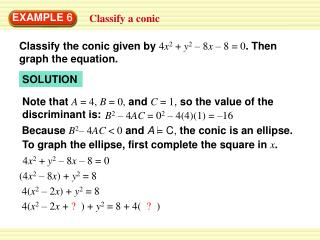DownloadDownload Presentation4( x 2 – 2 x + ? ) + y 2 = 8 + 4( ? )

# 4( x 2 – 2 x + ? ) + y 2 = 8 + 4( ? )

Télécharger la présentation## 4( x 2 – 2 x + ? ) + y 2 = 8 + 4( ? )

- - - - - - - - - - - - - - - - - - - - - - - - - - - E N D - - - - - - - - - - - - - - - - - - - - - - - - - - -
##### Presentation Transcript

1. Note thatA = 4, B = 0,andC = 1, so the value of the discriminant is: B2 – 4AC = 02 – 4(4)(1) = –16 BecauseB2– 4AC < 0andA = C, the conic is an ellipse. To graph the ellipse, first complete the square in x. EXAMPLE 6 Classify a conic Classify the conic given by4x2 + y2 – 8x – 8 = 0. Then graph the equation. SOLUTION 4x2 + y2 – 8x – 8 = 0 (4x2 – 8x) + y2 = 8 4(x2 – 2x) + y2 = 8 4(x2– 2x + ? ) + y2 = 8 + 4( ? )

2. (x – 1)2 y2 12 + = 1 3 From the equation, you can see that(h, k) = (1, 0), a = 12 = 2 3 ,andb = 3. Use these facts to draw the ellipse. EXAMPLE 6 Classify a conic 4(x2– 2x + 1) + y2 = 8 + 4(1) 4(x – 1)2 + y2 = 12

3. EXAMPLE 7 Solve a multi-step problem Physical Science In a lab experiment, you record images of a steel ball rolling past a magnet. The equation 16x2 – 9y2 – 96x + 36y – 36 = 0 models the ball’s path. • What is the shape of the path? • Write an equation for the path in standard form. • Graph the equation of the path.

4. EXAMPLE 7 Solve a multi-step problem SOLUTION STEP 1 Identify the shape. The equation is a general second-degree equation withA = 16, B = 0,andC = –9. Find the value of the discriminant. B2 – 4AC = 02 – 4(16)(–9) = 576 BecauseB2 – 4AC > 0, the shape of the path is a hyperbola.

5. (x – 3)2 9 (y –2)216 – = 1 EXAMPLE 7 Solve a multi-step problem STEP 2 Write an equation. To write an equation of the hyperbola, complete the square in both xand ysimultaneously. 16x2 – 9y2 – 96x + 36y – 36 = 0 (16x2 – 96x) – (9y2 – 36y) = 36 16(x2– 6x + ? ) – 9(y2– 4y + ? ) = 36 + 16( ? ) – 9(?) 16(x2– 6x + 9) – 9(y2– 4y + 4) = 36 + 16(9) – 9(4) 16(x – 3)2 – 9(y – 2)2 = 144

6. Graph the equation. From the equation, the transverse axis is horizontal, (h, k) = (3, 2), a = 9 = 3 andb = 16. = 4 EXAMPLE 7 Solve a multi-step problem STEP 3 The vertices are at(3 +a, 2),or(6, 2)and(0, 2).

7. EXAMPLE 7 Solve a multi-step problem STEP 3 Plot the center and vertices. Then draw a rectangle 2a = 6 units wide and 2b = 8 units high centered at (3, 2), draw the asymptotes, and draw the hyperbola. Notice that the path of the ball is modeled by just the right-hand branch of the hyperbola.

8. for Examples 6 and 7 GUIDED PRACTICE 10. Classify the conic given byx2 + y2 – 2x + 4y + 1 = 0. Then graph the equation. ANSWER (x – 1)2 +( y + 2)2 = 4

9. + y2 = 1 (x – 1)2 6 3 for Examples 6 and 7 GUIDED PRACTICE 11. Classify the conic given by2x2 + y2 – 4x – 4= 0. Then graph the equation. ANSWER Circle

10. for Examples 6 and 7 GUIDED PRACTICE 12. Classify the conic given byy2 – 4y2 – 2x + 6= 0. Then graph the equation. ANSWER Parabola (y – 2)2 = 2(x –1)

11. (x –2)2 (y +2)2 = 1 4 16 for Examples 6 and 7 GUIDED PRACTICE 13. Classify the conic given by4x2 – y2 – 16x –4y – 4= 0. Then graph the equation. ANSWER Hyperbola

12. y2 – = 1 4 4(x – 1.5)2 4 for Examples 6 and 7 GUIDED PRACTICE 14. Astronomy An asteroid’s path is modeled by 4x2 + 6.25y2 – 12x –16 = 0 where x and y are in astronomical units from the sun. Classify the path and write its equation in standard form. Then graph the equation. ANSWER Hyperbola